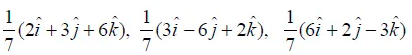NCERT Solutions for Maths Class 12 Exercise 10.3

# NCERT Solutions for Maths Class 12 Exercise 10.3

NCERT Solutions for Maths Class 12 Exercise 10.3

Hello Students. Welcome to maths-formula.com. In this post, you will find the complete NCERT Solutions for Maths Class 12 Exercise 10.3.

You can download the PDF of NCERT Books Maths Chapter 10 for your easy reference while studying NCERT Solutions for Maths Class 12 Exercise 10.3.

Class 12th is a very crucial stage of your student’s life, since you take all important decisions about your career on this stage. Mathematics plays a vital role to take decision for your career because if you are good in mathematics, you can choose engineering and technology field as your career.

NCERT Solutions for Maths Class 12 Exercise 10.3 helps you to solve each and every problem with step by step explanation which makes you strong in mathematics.

All the schools affiliated with CBSE, follow the NCERT books for all subjects. You can check your syllabus from NCERT Syllabus for Mathematics Class 12.

NCERT Solutions for Maths Class 12 Exercise 10.3 are prepared by the experienced teachers of CBSE board. If you are preparing for JEE Mains and NEET level exams, then it will definitely make your foundation strong.

If you want to recall All Maths Formulas for Class 12, you can find it by clicking this link.

If you want to recall All Maths Formulas for Class 11, you can find it by clicking this link.

NCERT Solutions for Maths Class 12 Exercise 10.1

NCERT Solutions for Maths Class 12 Exercise 10.3

Maths Class 12 Ex 10.3 Question 1.

Find the angle between two vectors a and b with magnitudes √3 and 2, respectively, having a . b = √6.

Solution:

Let θ be the angle between two vectors.

Given that a = √3, b = 2 and a . b = √6

Maths Class 12 Ex 10.3 Question 2.

Solution:

Let θ be the angle between a and b.

Maths Class 12 Ex 10.3 Question 3.

Find the projection of the vector ij on the vector i + j.

Solution:

Maths Class 12 Ex 10.3 Question 4.

Find the projection of the vector i + 3j + 7k on the vector 7i j + 8k.

Solution:

Maths Class 12 Ex 10.3 Question 5.

Show that each of the given three vectors is a unit vector.Also, show that they are mutually perpendicular to each other.

Solution:

Maths Class 12 Ex 10.3 Question 6.

Find | | and | |, if (a + b).(ab) = 8 | |.

Solution:

Maths Class 12 Ex 10.3 Question 7.

Solution:

Maths Class 12 Ex 10.3 Question 8.

Find the magnitude of two vectors a and b, having the same magnitude and such that the angle between them is 60° and their scalar product is ½.

Solution:
We have given that, |a| = |b|, θ = 60˚ and a.b = ½

We know that

Maths Class 12 Ex 10.3 Question 9.

Solution:

Given that, a is a unit vector, then |a| = 1.

Maths Class 12 Ex 10.3 Question 10.

If a = 2i + 2j + 3k, b = -i + 2j + k and c = 3i + j are such that a + λb is perpendicular to c, then find the value of λ.

Solution:

Maths Class 12 Ex 10.3 Question 11.

Show that |a|b + |b|a is perpendicular to |a|b – |b|a, for any two non-zero vectors a and b.

Solution:

Maths Class 12 Ex 10.3 Question 12.

Solution:

This implies that, b = 0
Hence, b is any vector.

Maths Class 12 Ex 10.3 Question 13.

Solution:

Maths Class 12 Ex 10.3 Question 14.

Solution:

Maths Class 12 Ex 10.3 Question 15.

If the vertices A, B, C of a triangle ABC are (1, 2, 3), (-1, 0, 0), (0, 1, 2), respectively, then find ABC. [ABC is the angle between the vectors BA and BC.]

Solution:

Maths Class 12 Ex 10.3 Question 16.

Show that the points A(1, 2, 7), B(2, 6, 3) and C(3, 10, -1) are collinear.

Solution:

The position vectors of points A, B and C are:

Maths Class 12 Ex 10.3 Question 17.

Solution:

The position vectors of the points A, B and C are:

Maths Class 12 Ex 10.3 Question 18.

If a is a non-zero vector of magnitude ‘a’ and λ is a non-zero scalar, then λa is unit vector if
(A) λ = 1
(B) λ = – 1
(C) a = |λ|
(D) a1/|λ|

Solution:

Hence, the correct answer is option (D).